# The Straight Line Exercise 3(e) Solutions Inter Maths 1b

The Straight Line Exercise 3(e) Maths 1b textbook Chapter 3 Inter Solutions are given.

Study the textbook lesson The Straight Line very well.

All problems with answers are given. These are very simple to understand.

Observe the example problems and solutions given in the textbook. Try them well.

Observe the given solutions and try them in your own method.

You can also see

SSC Maths text book Solutions class 10

Inter Maths 1A Solutions textbook

Inter Maths 1B textbook solutions

Inter Maths 1IA text book solutions

Inter Maths IIB text book solutions

The Straight Line

Exercise 3(a)

Exercise 3(b)

Exercise 3(c)

Exercise 3(d)

M

# Maths Solutions for The Straight Line

Chapter 3 – The Straight Line

Exercise 3(e)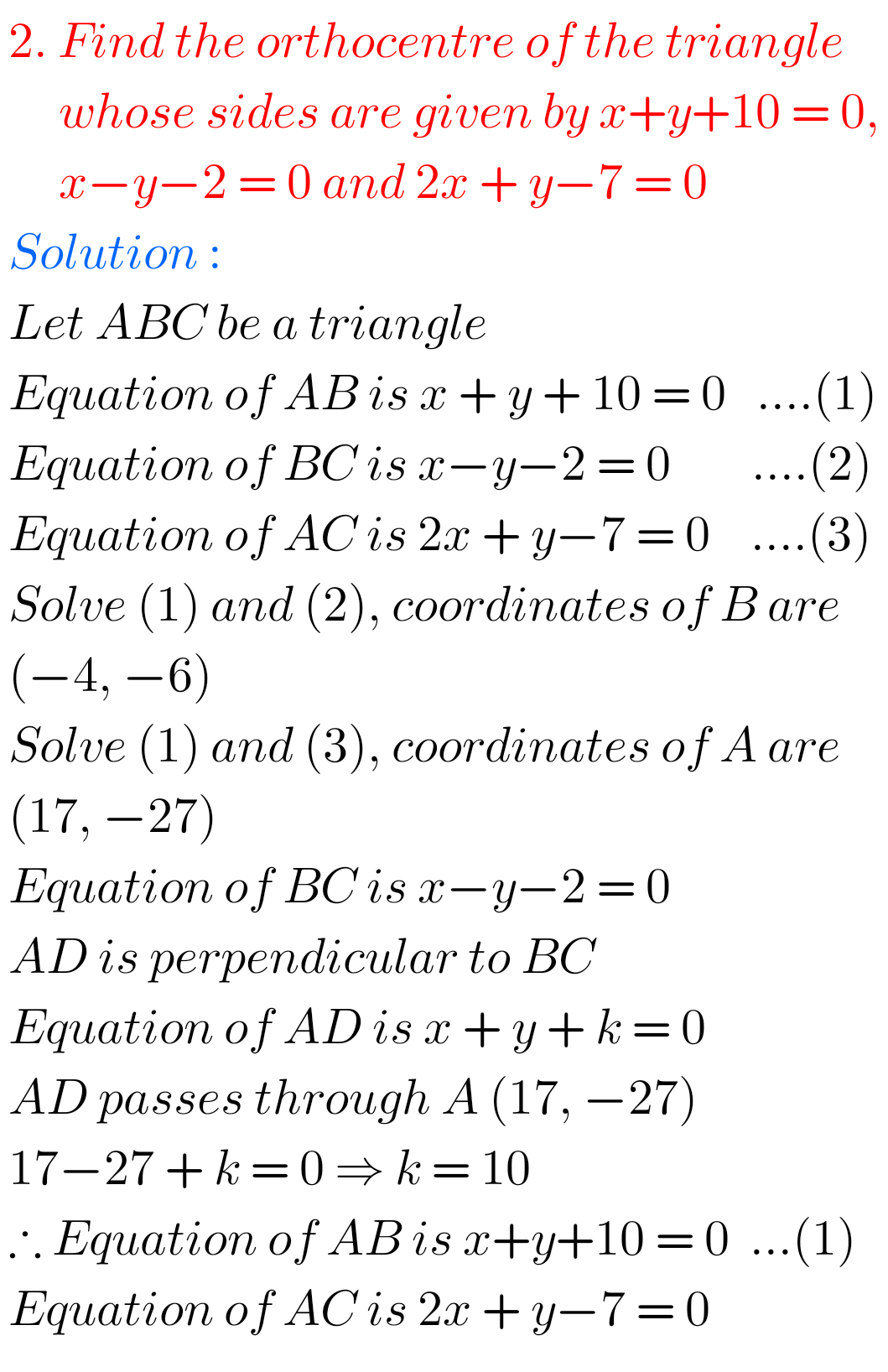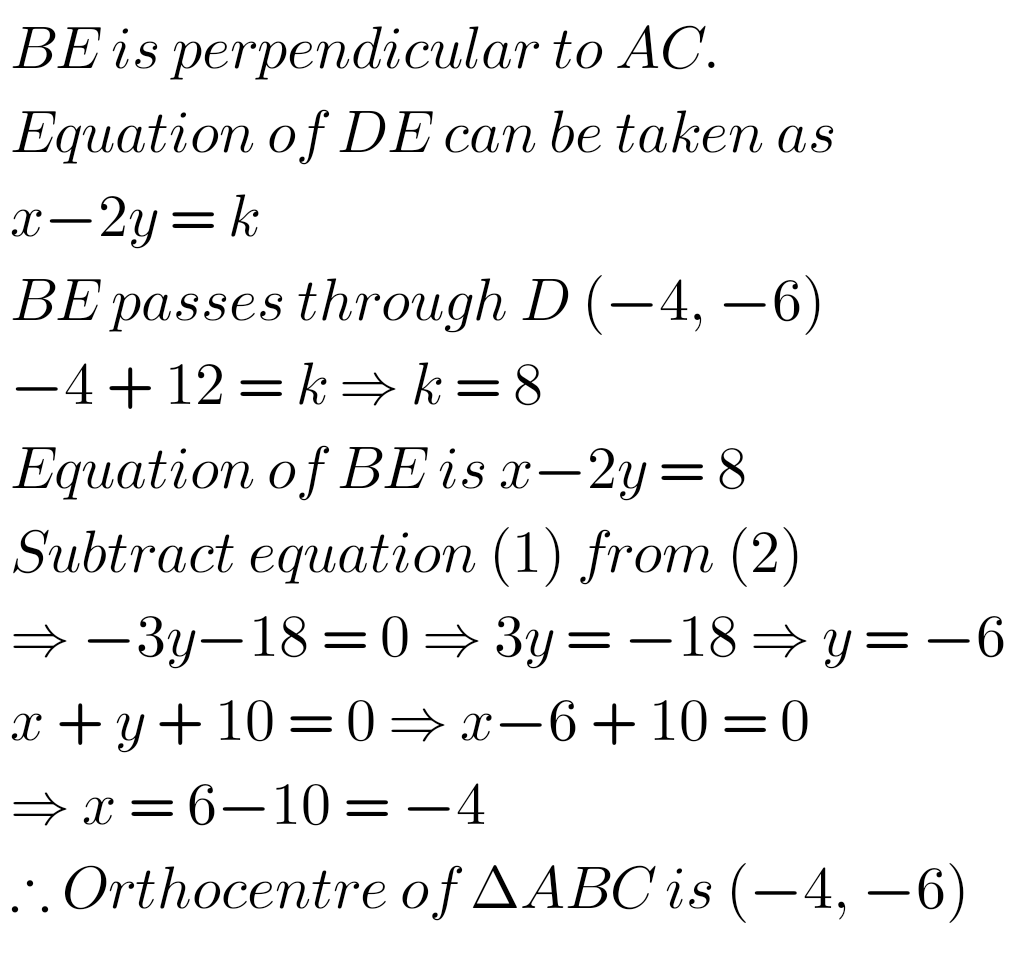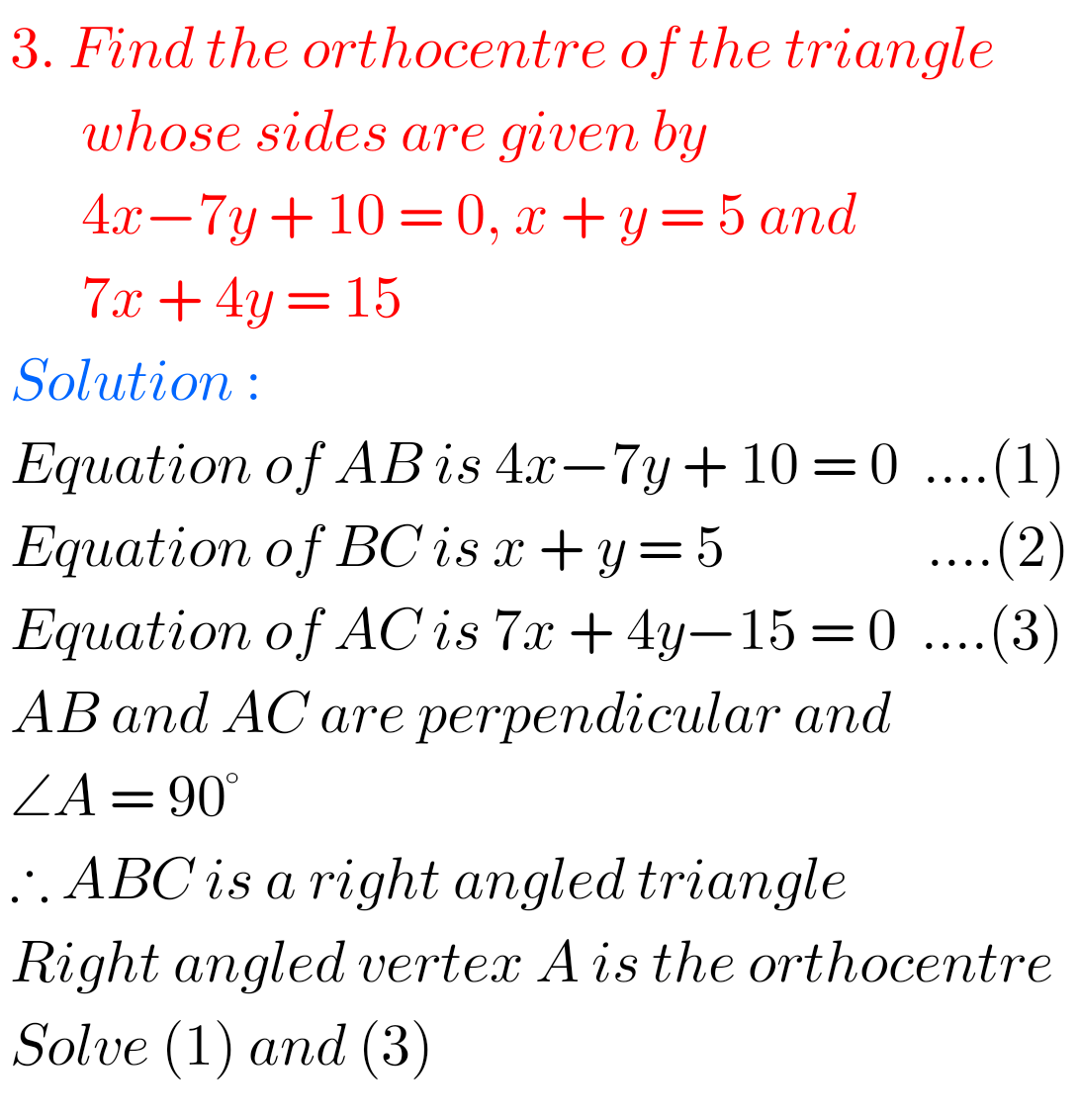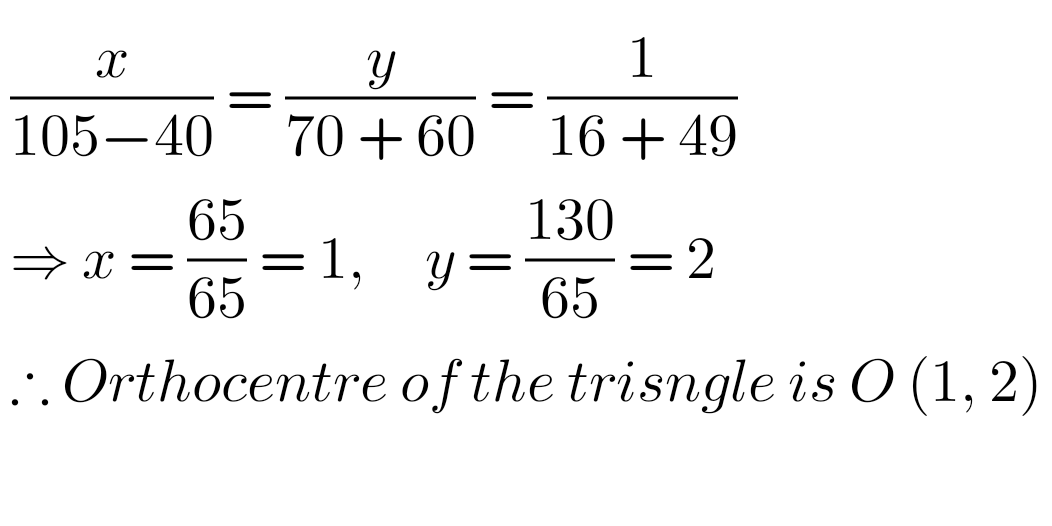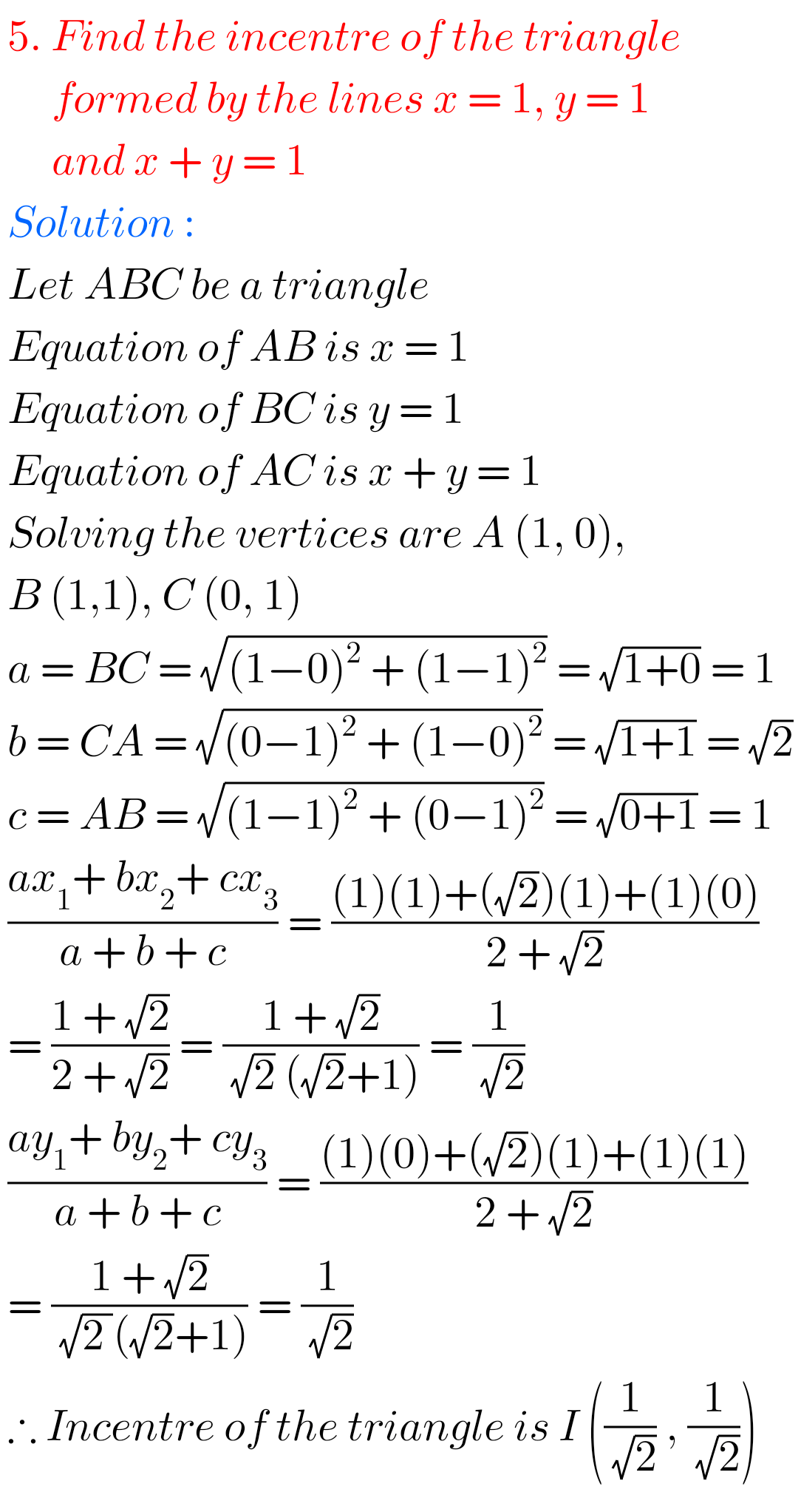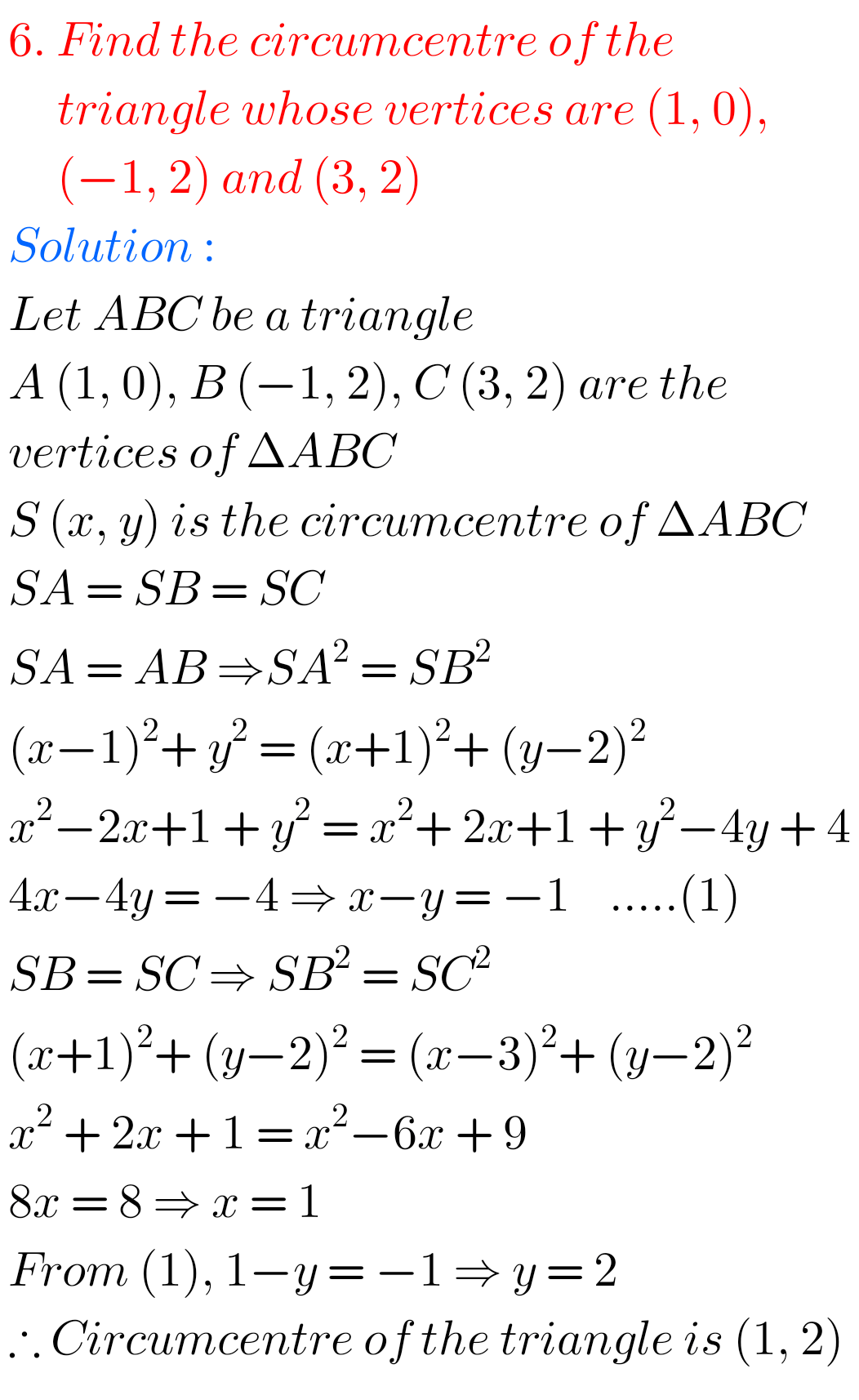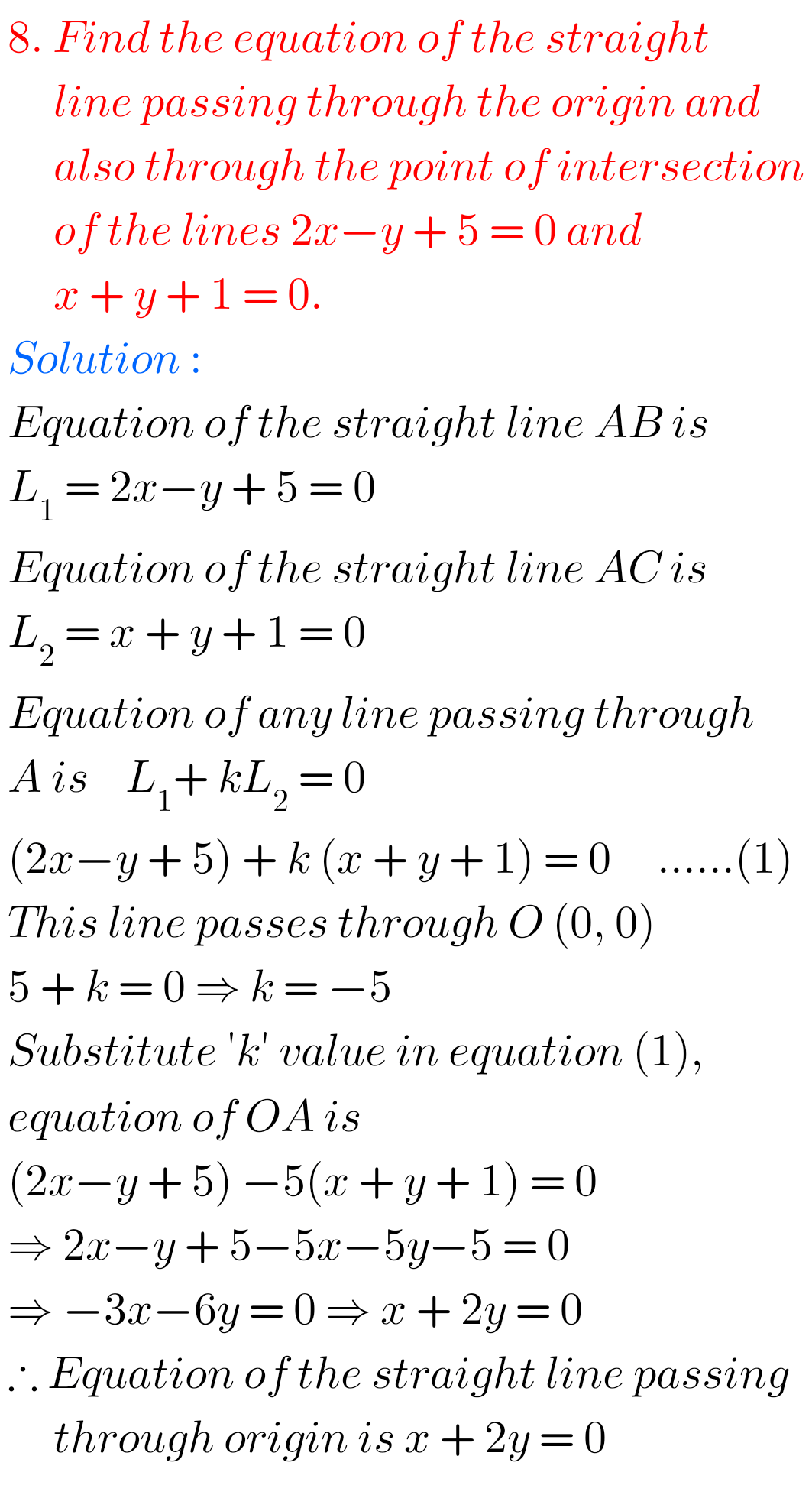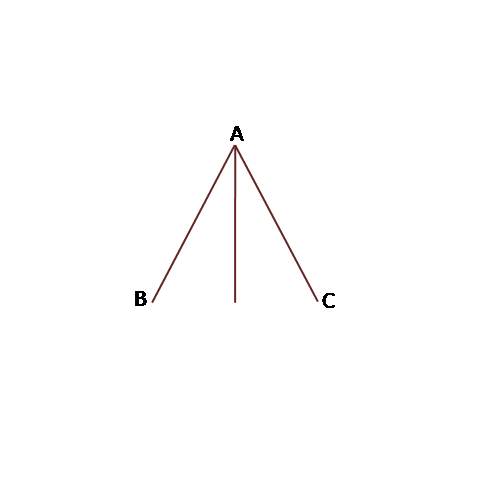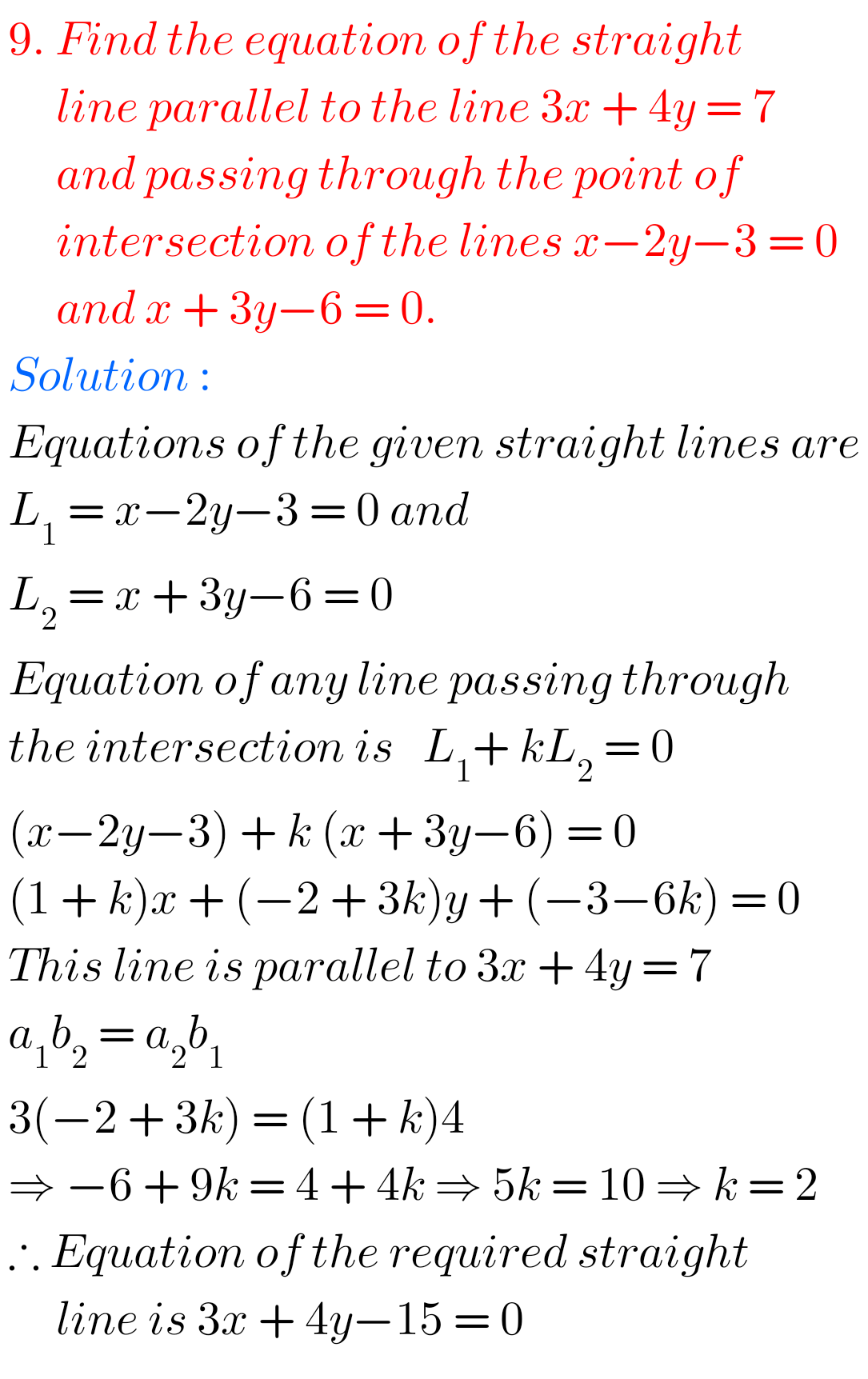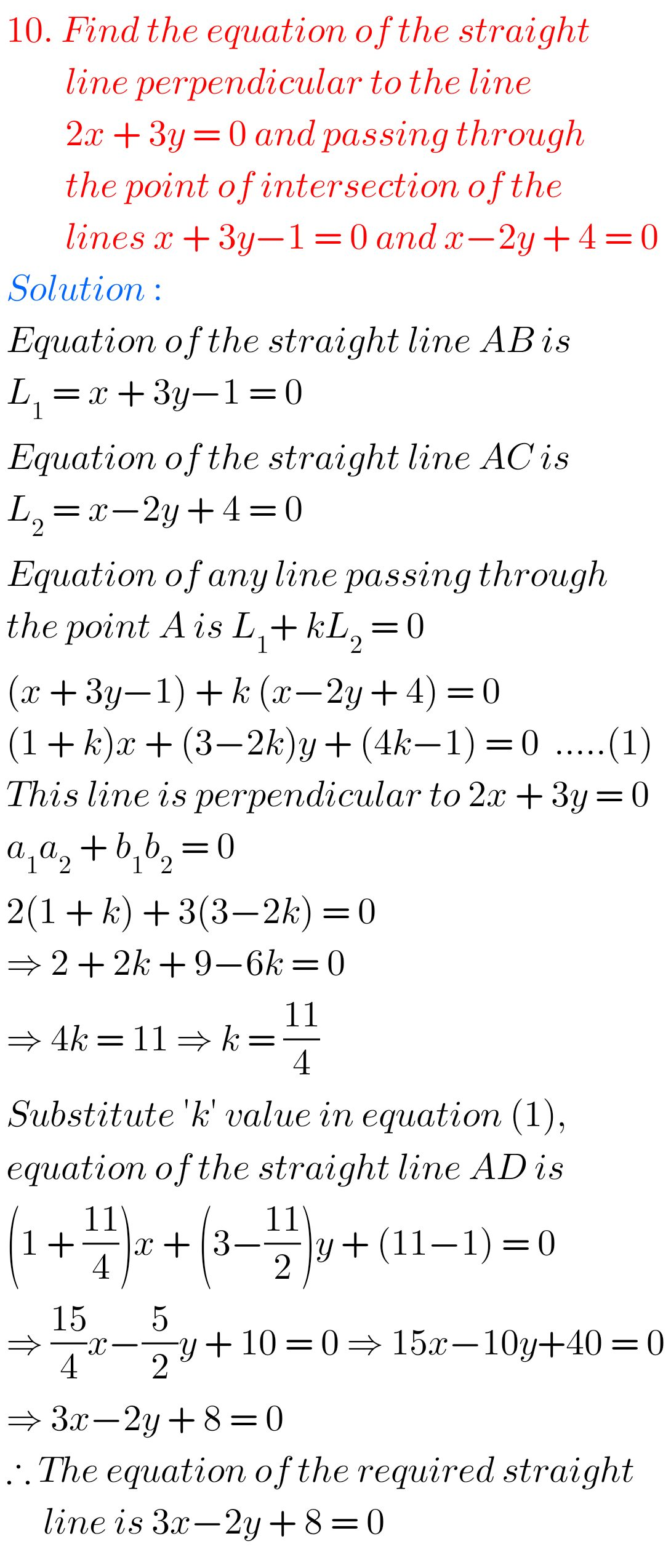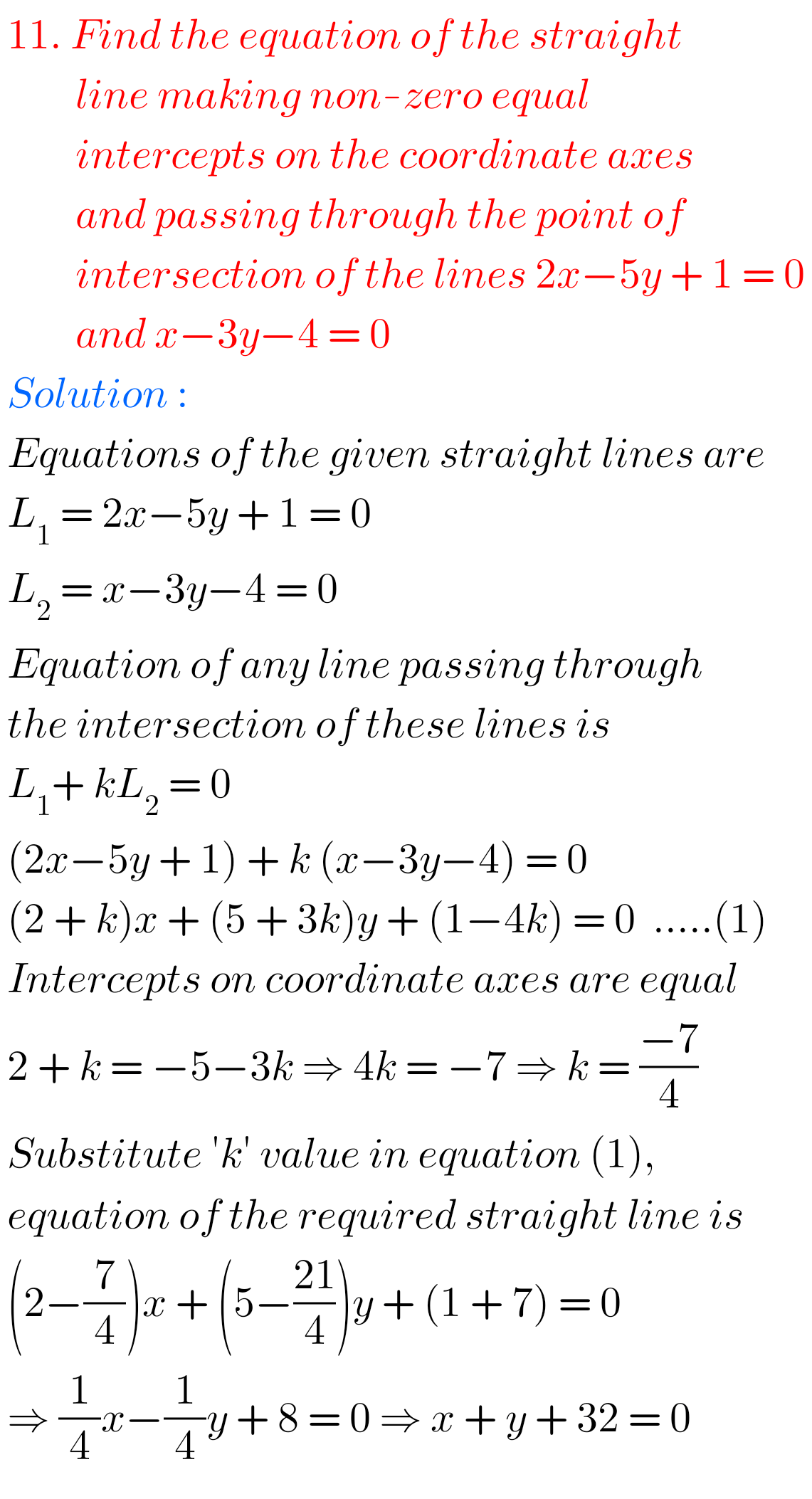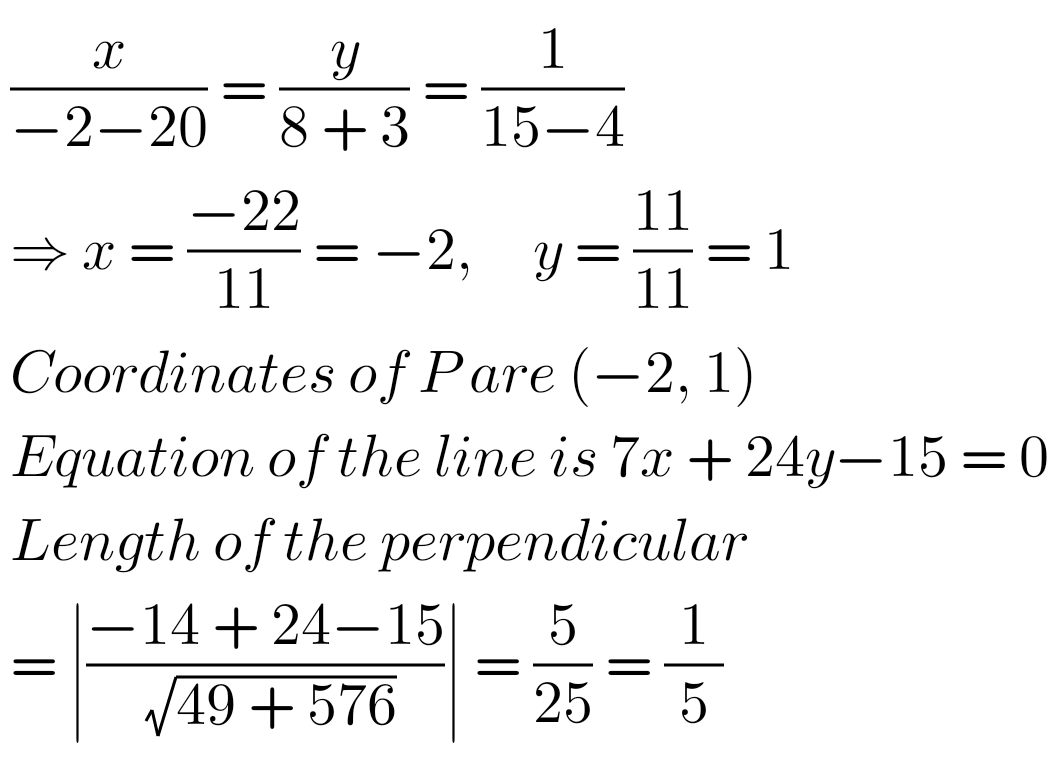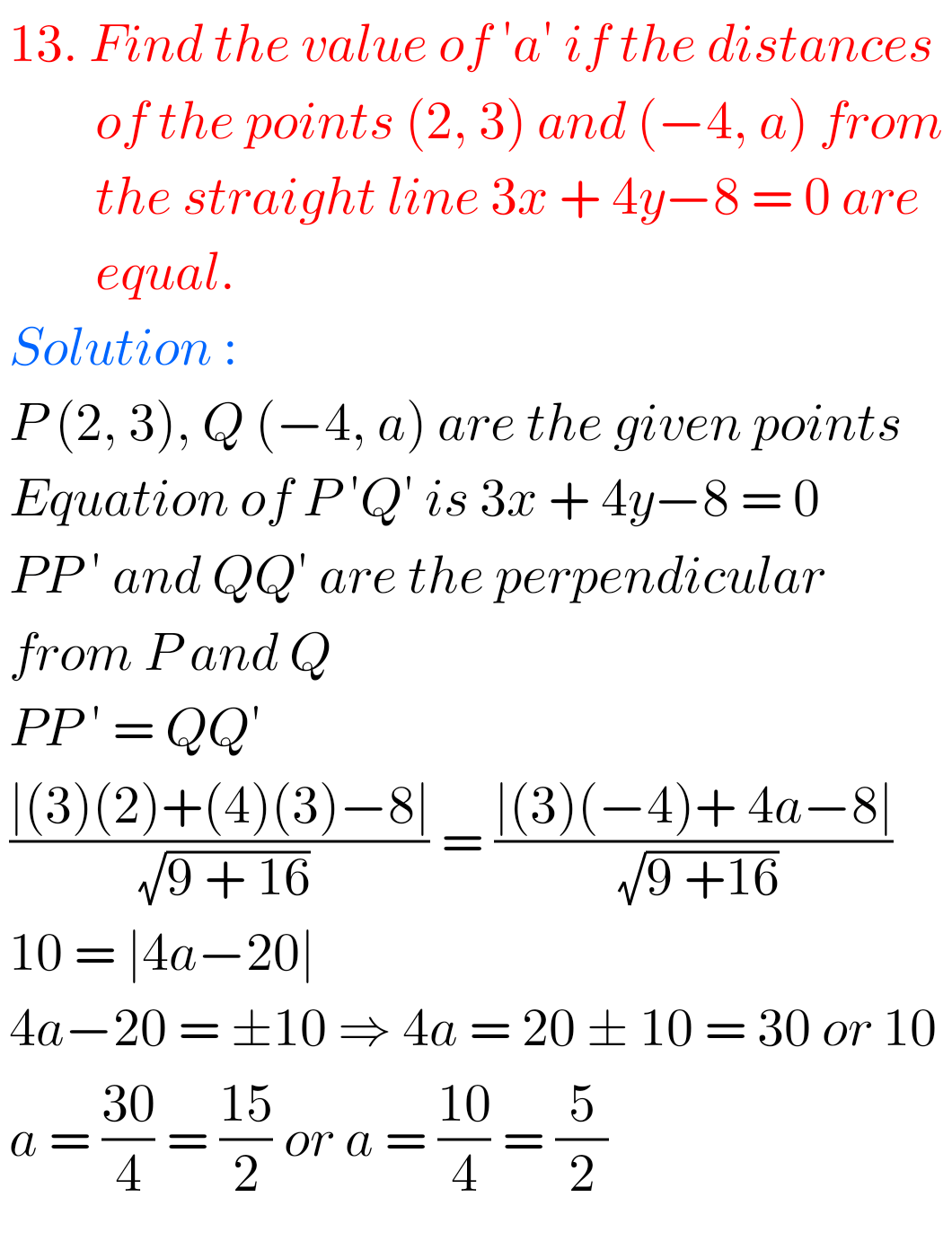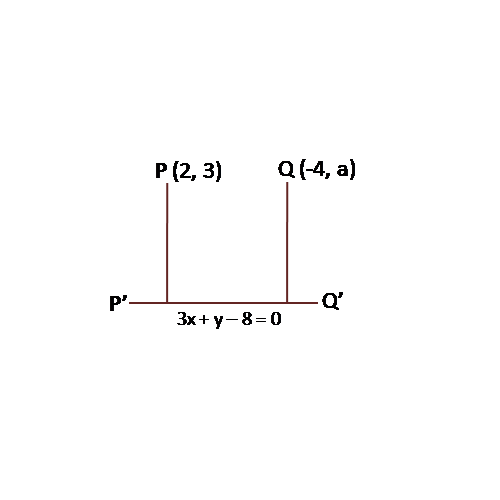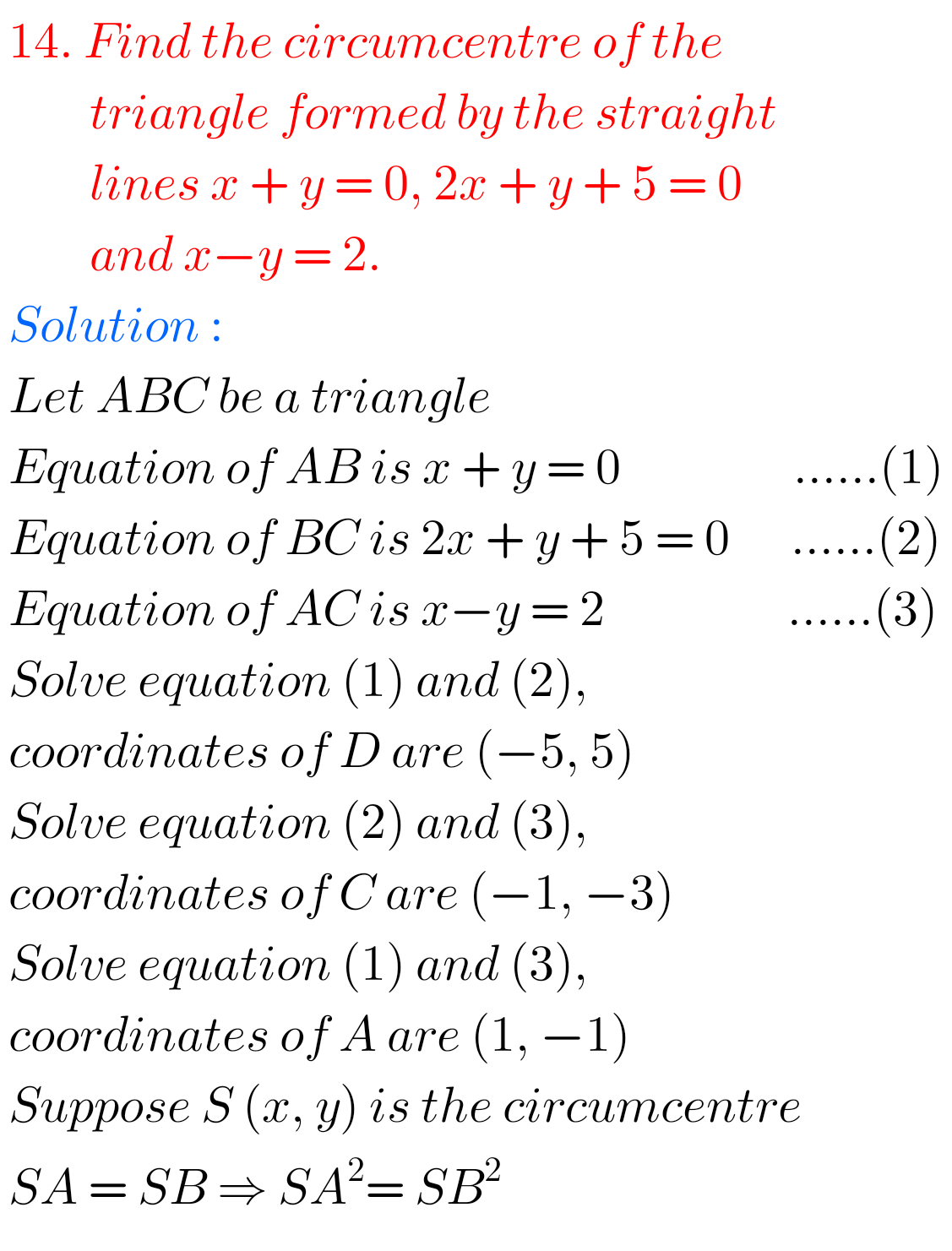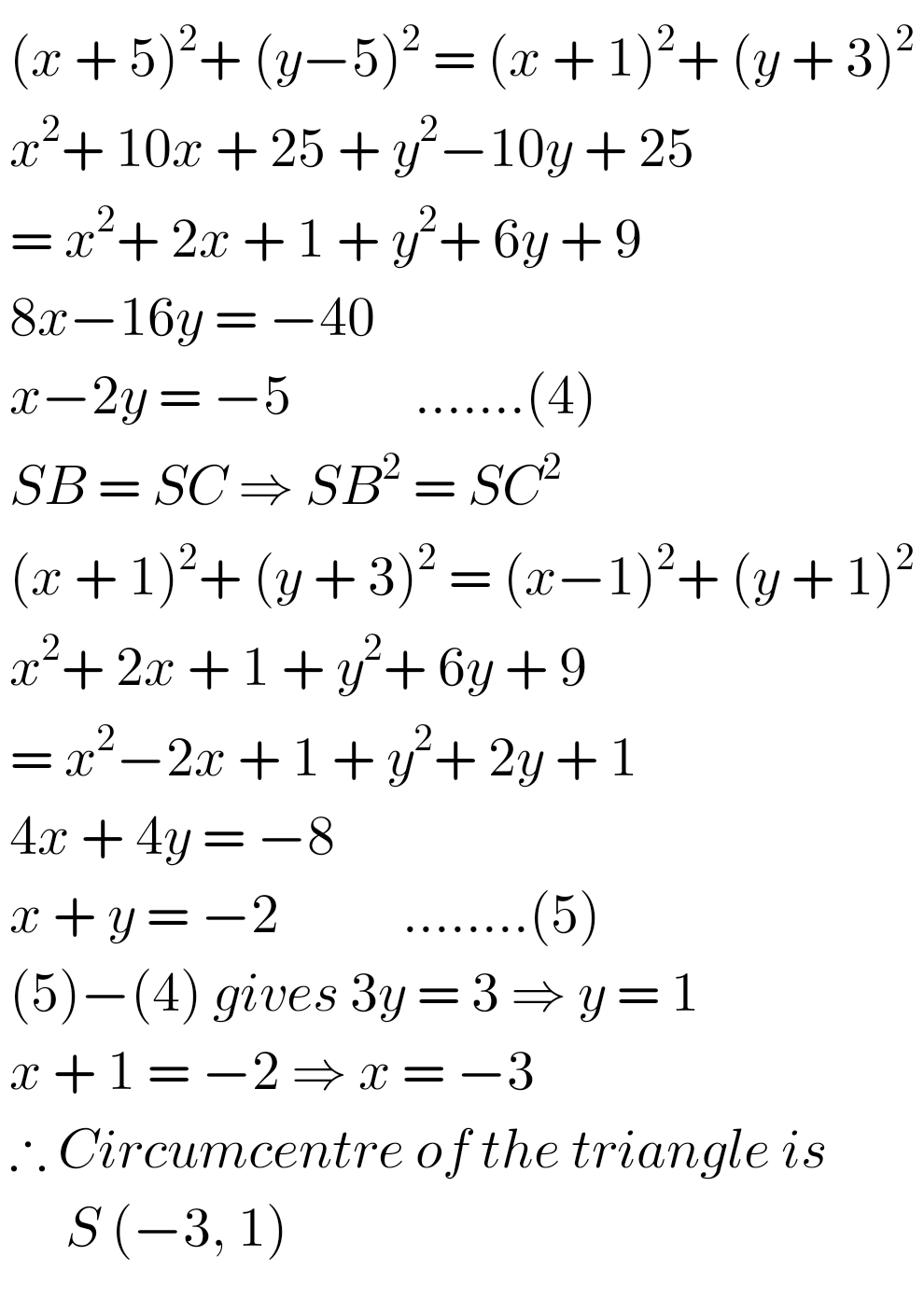## Solutions for exercise 3(e) Maths 1b Inter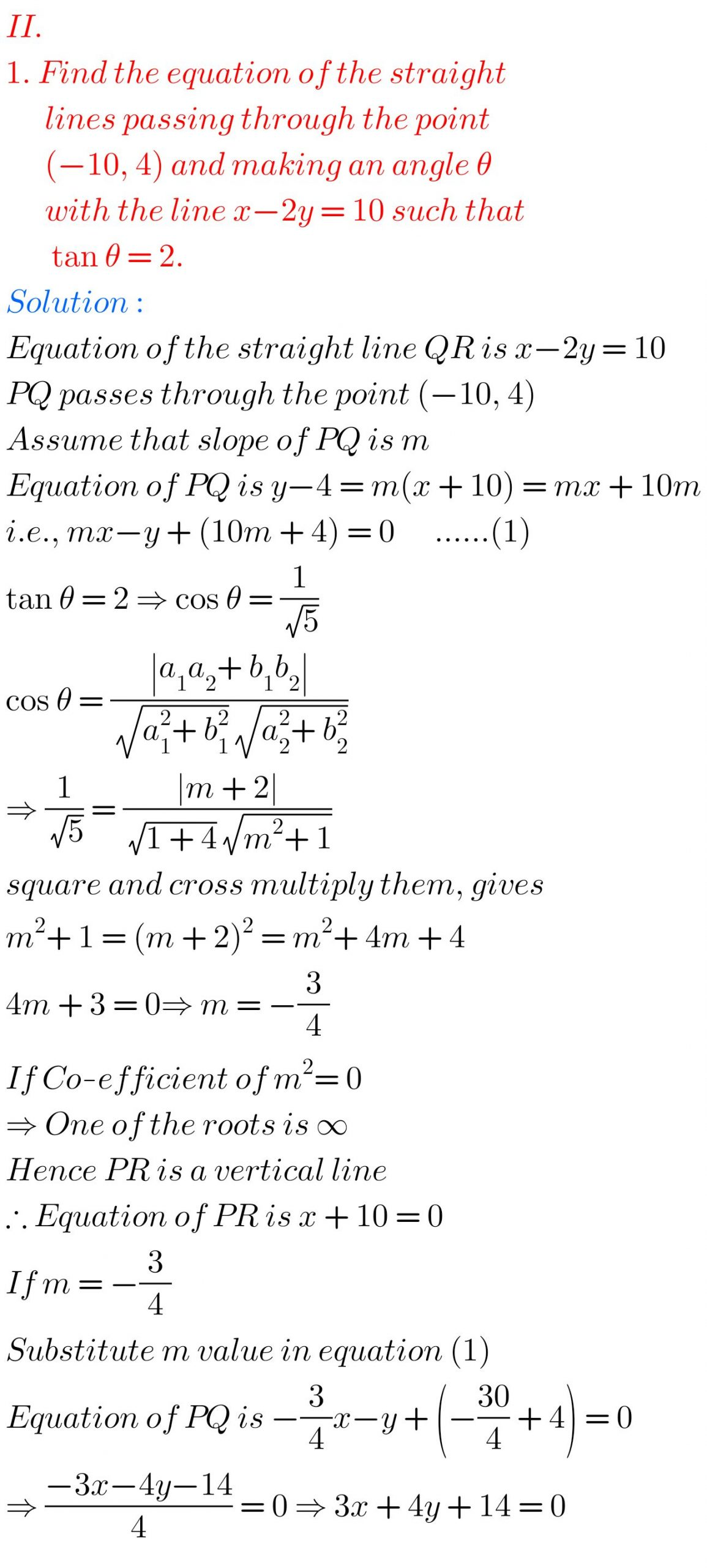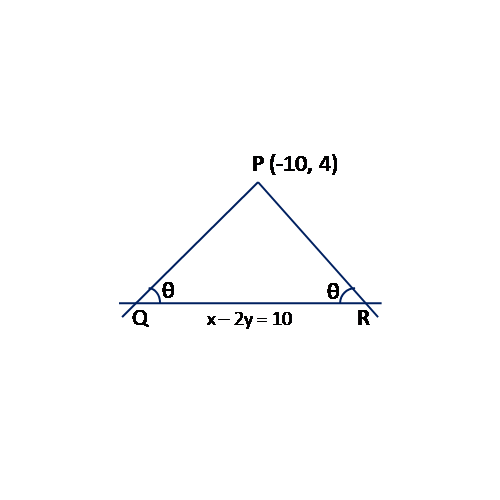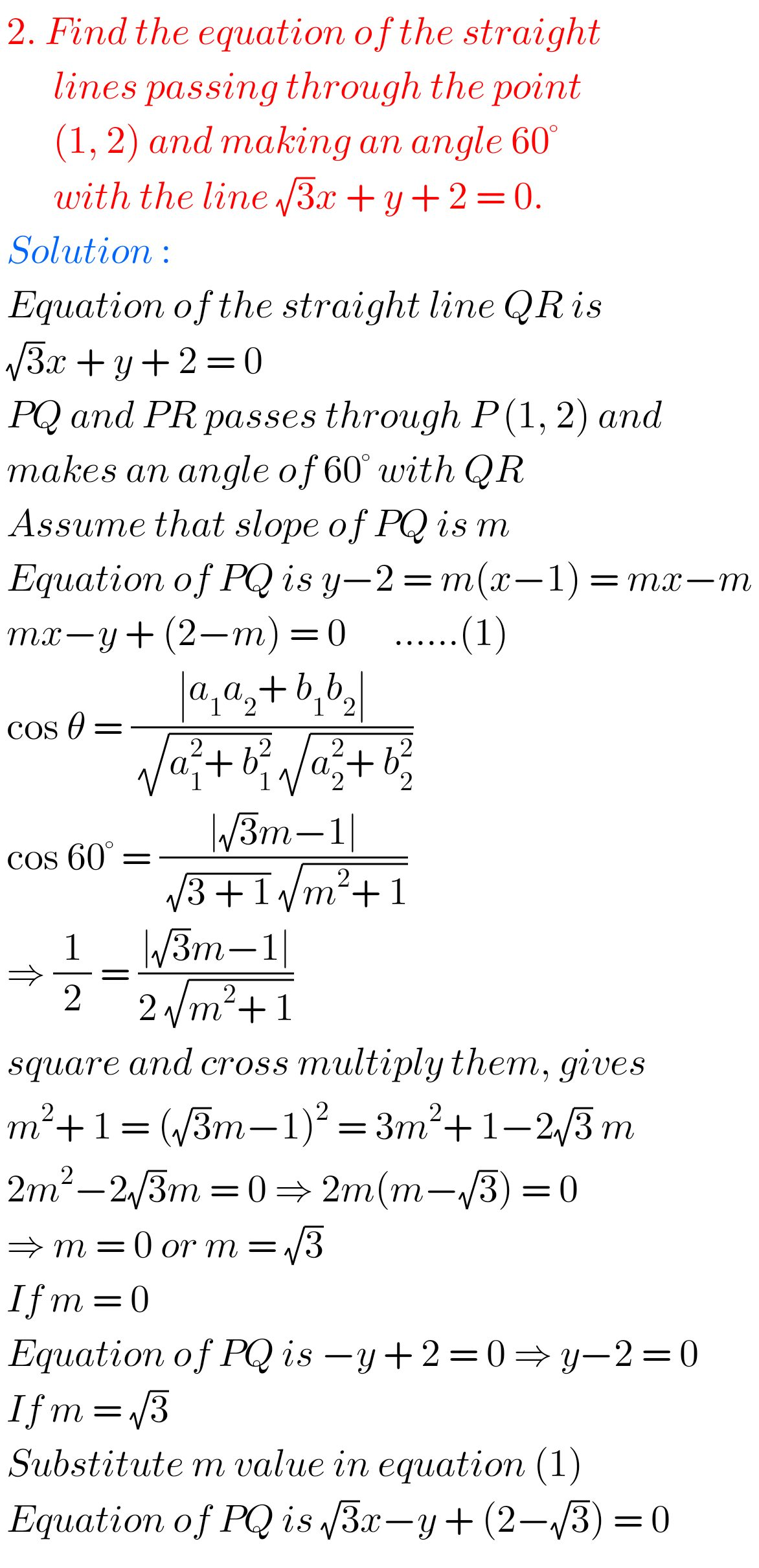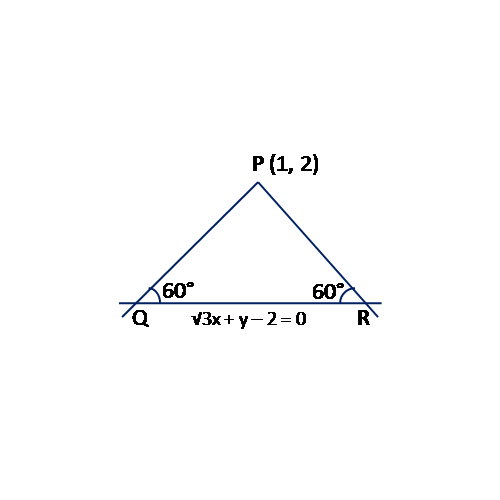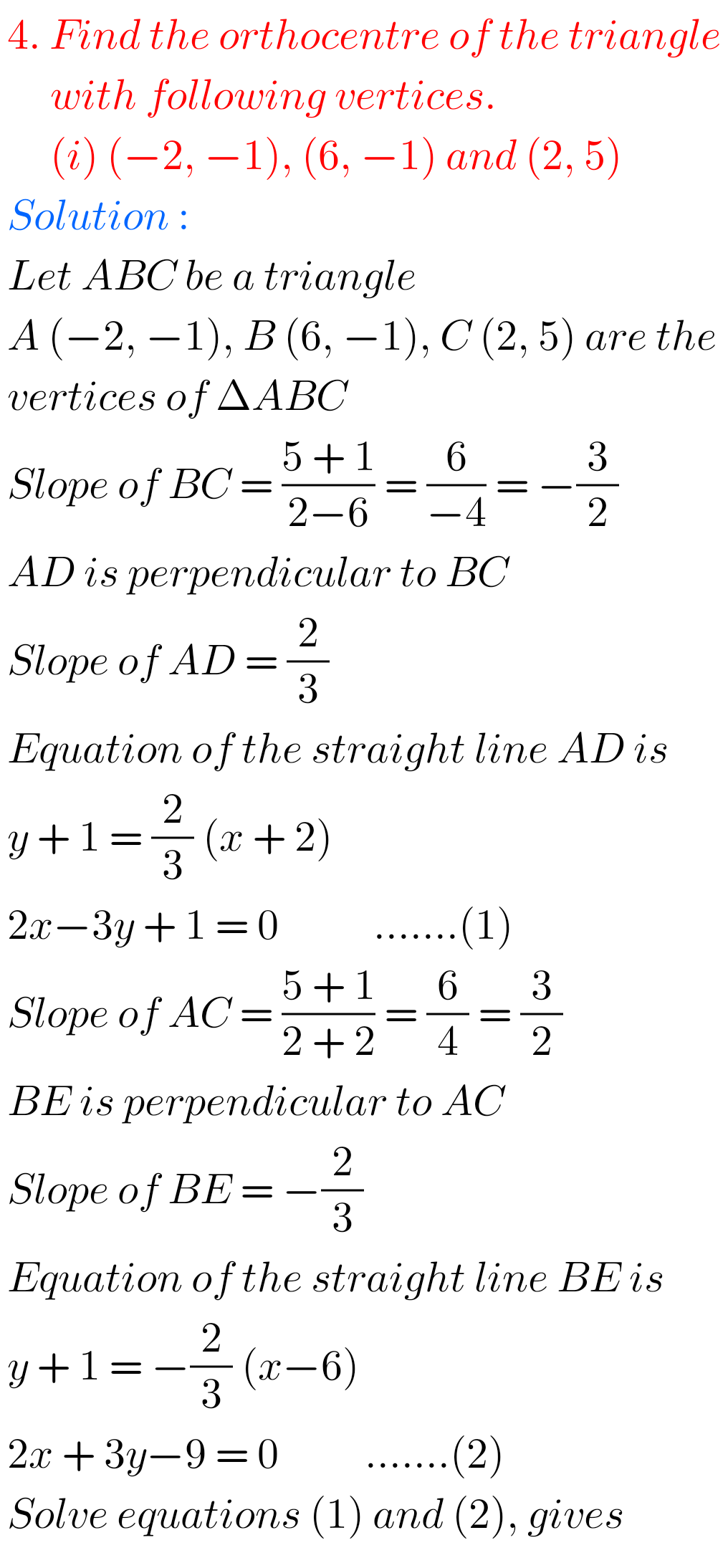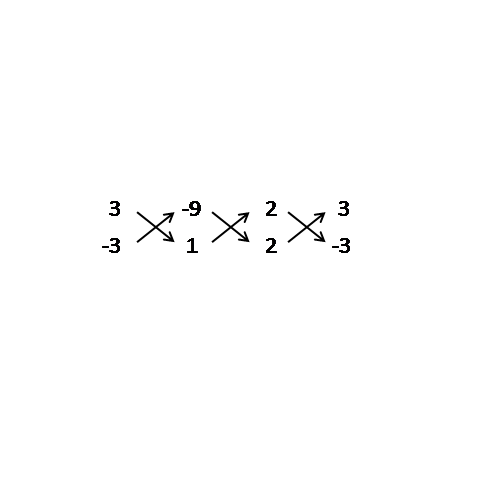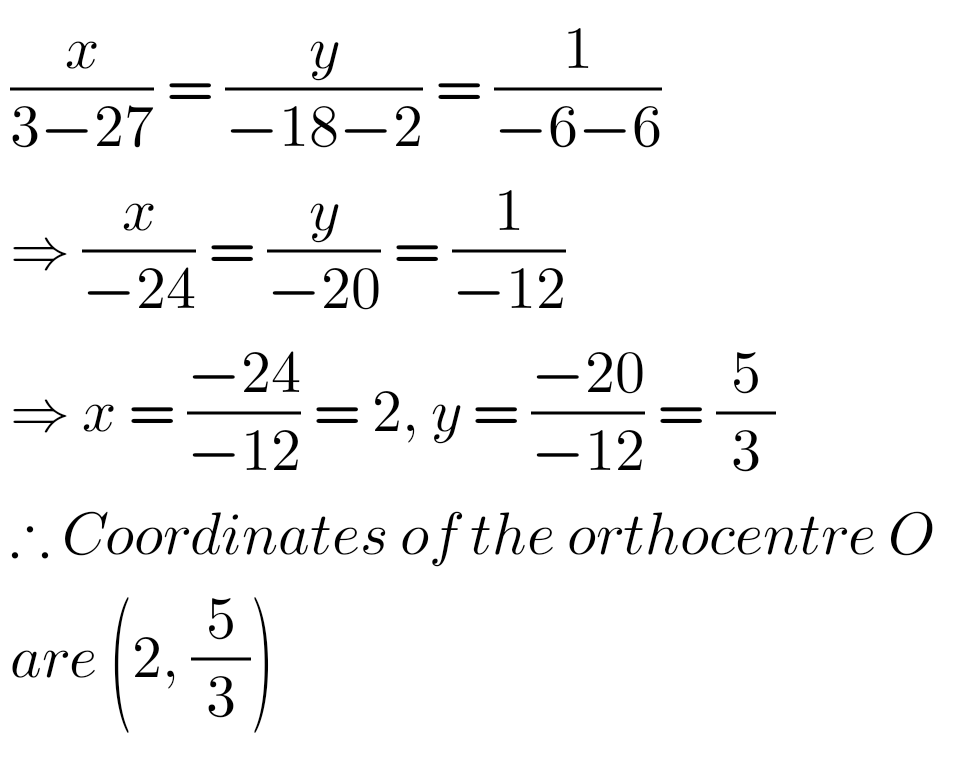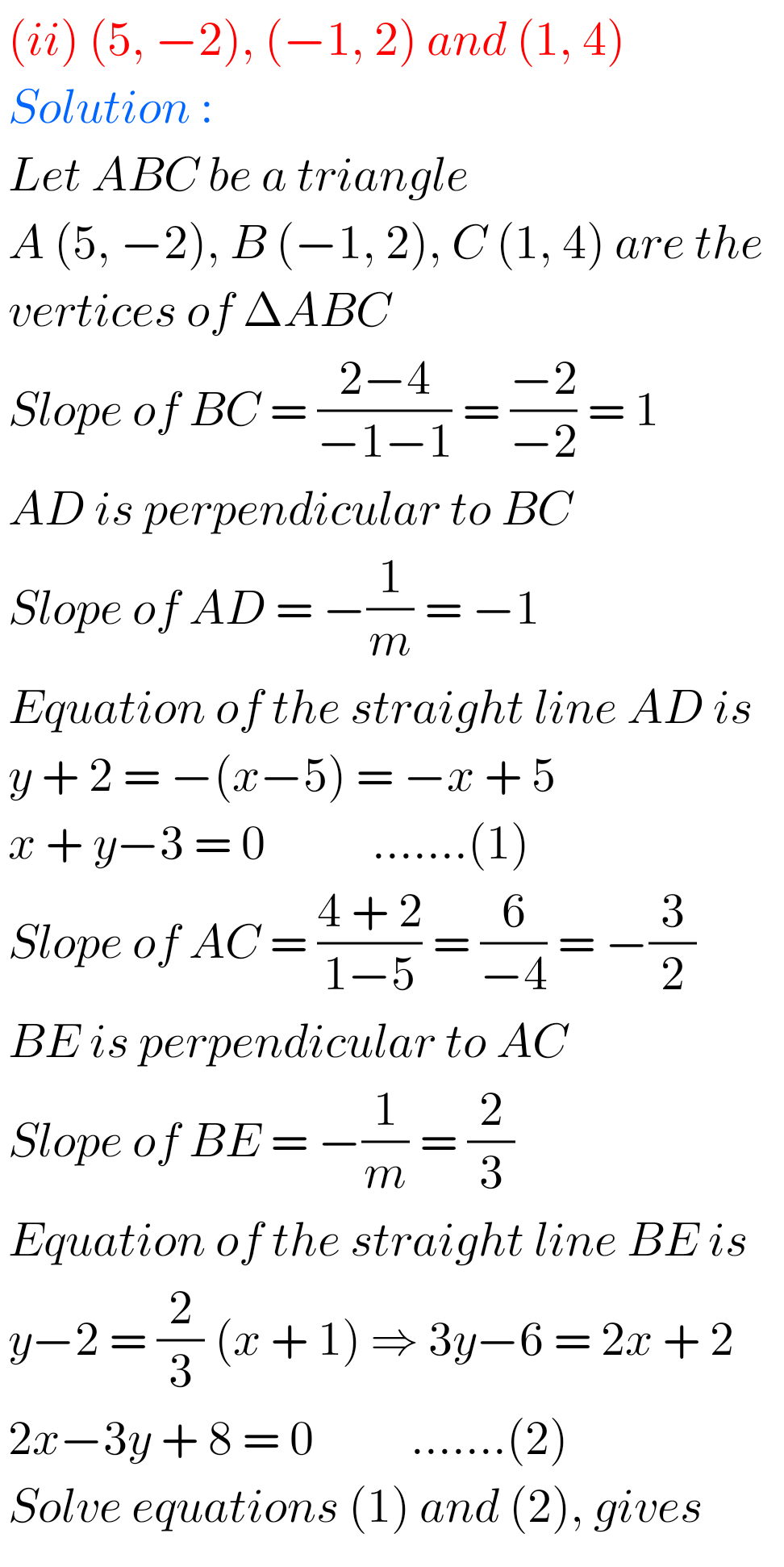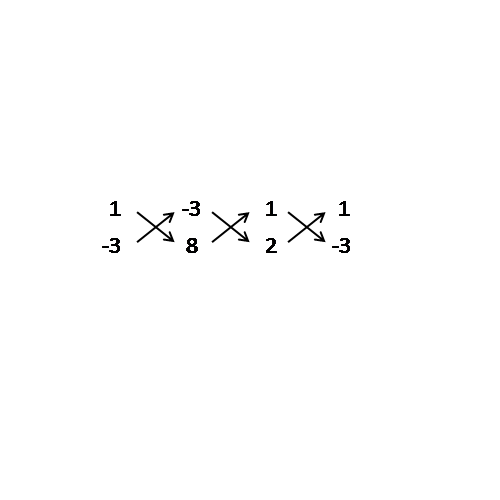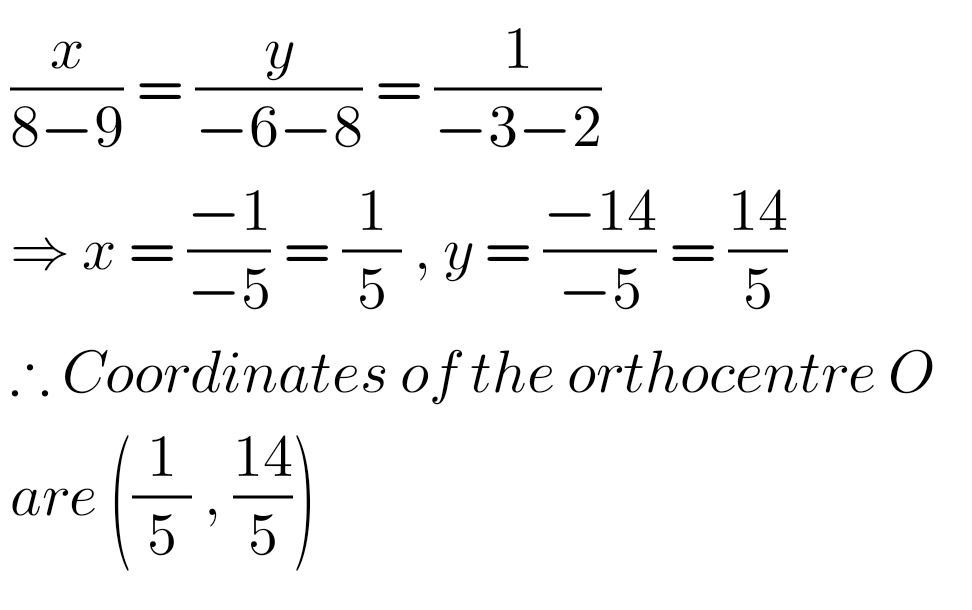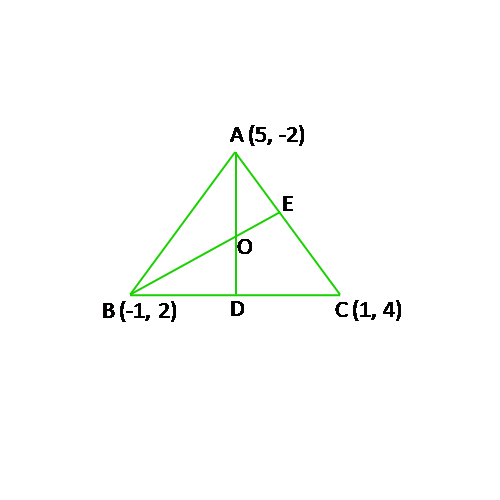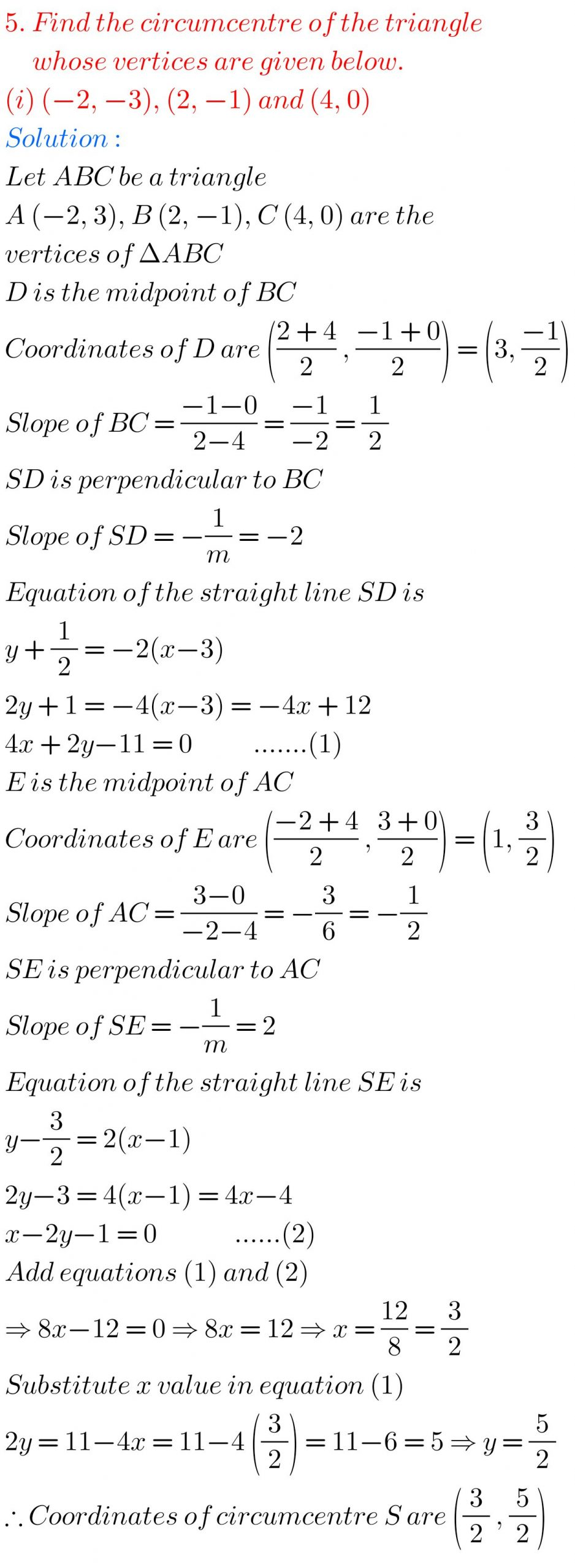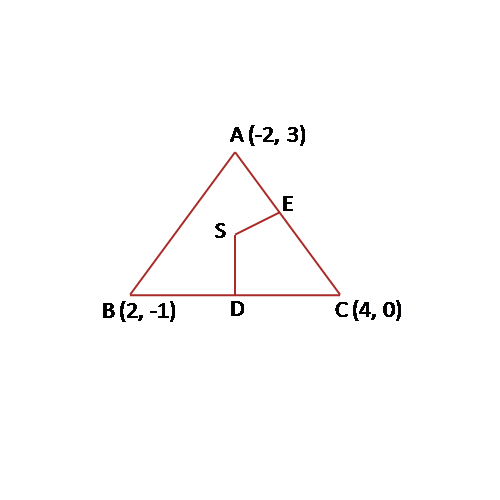F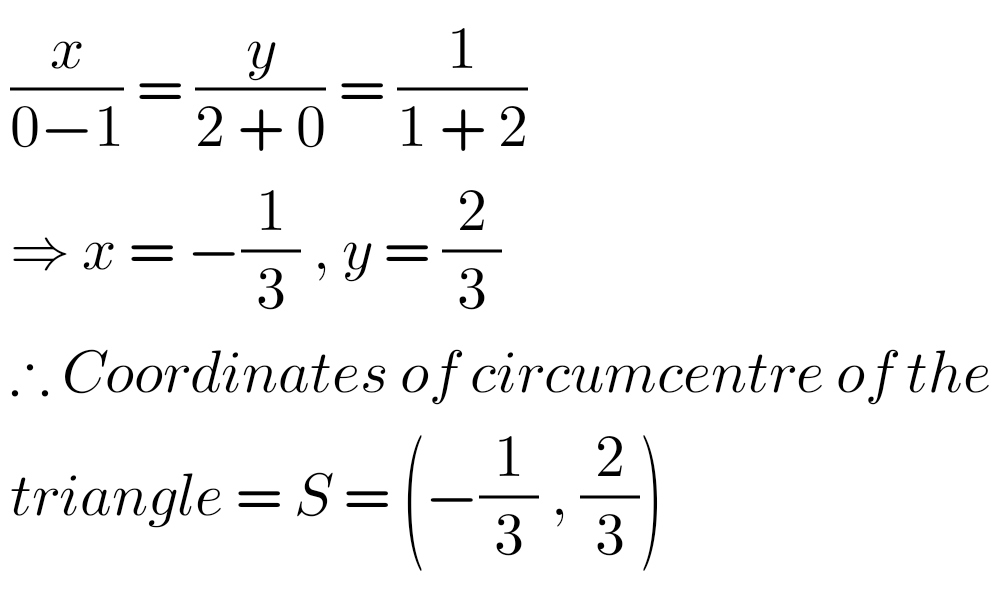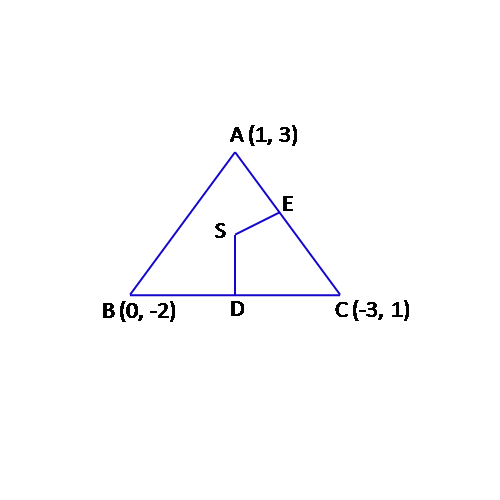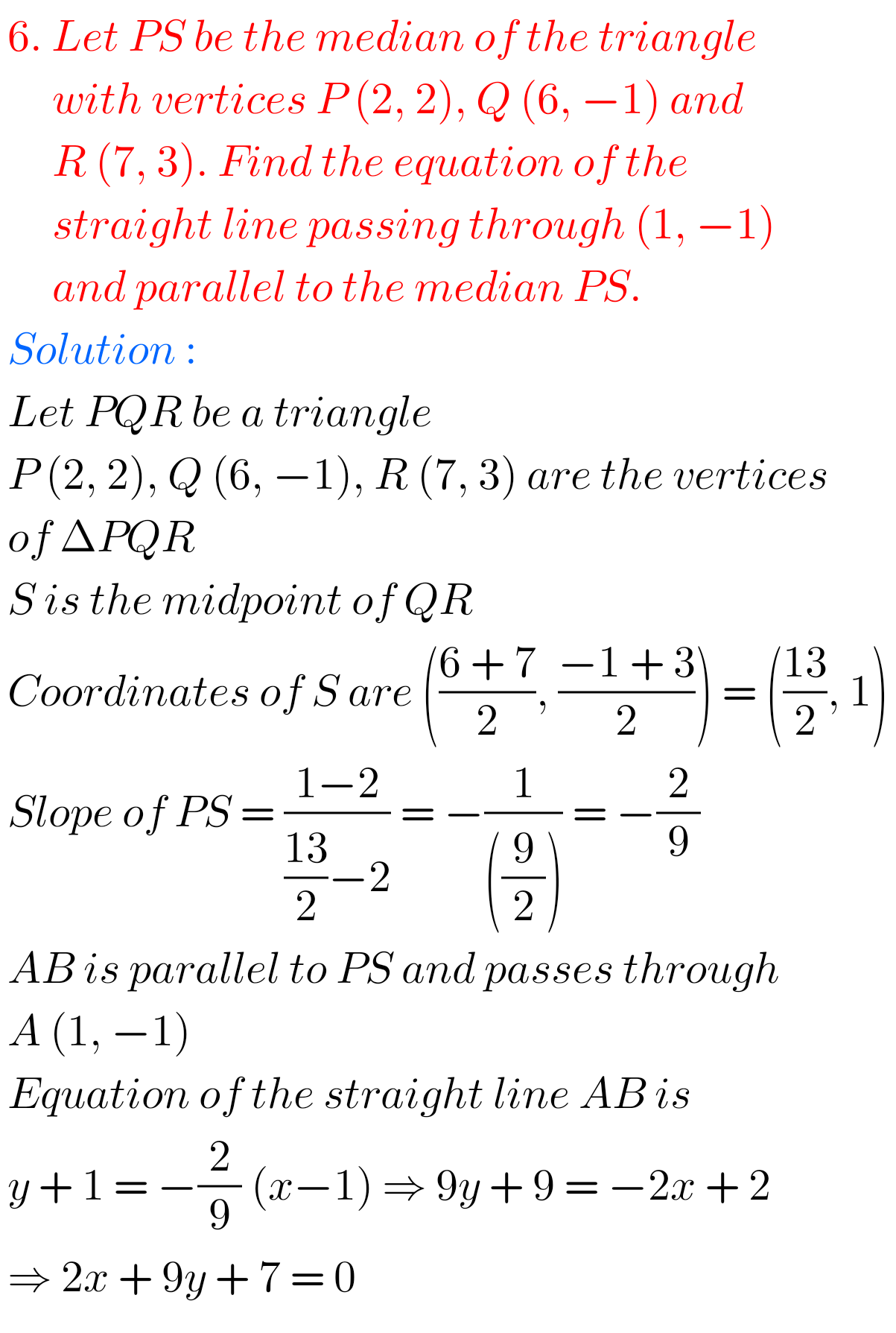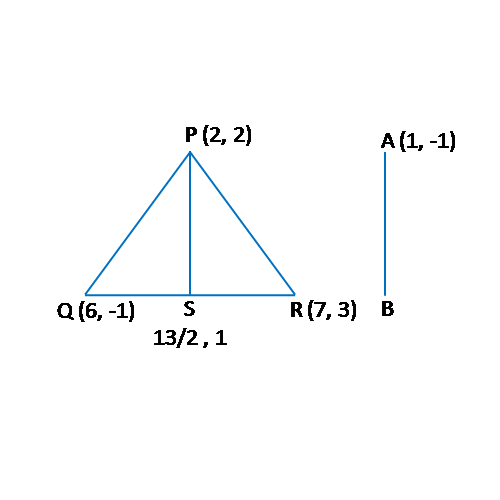## AP & TS Inter Maths Solutions for The Straight Line exercise 3(e)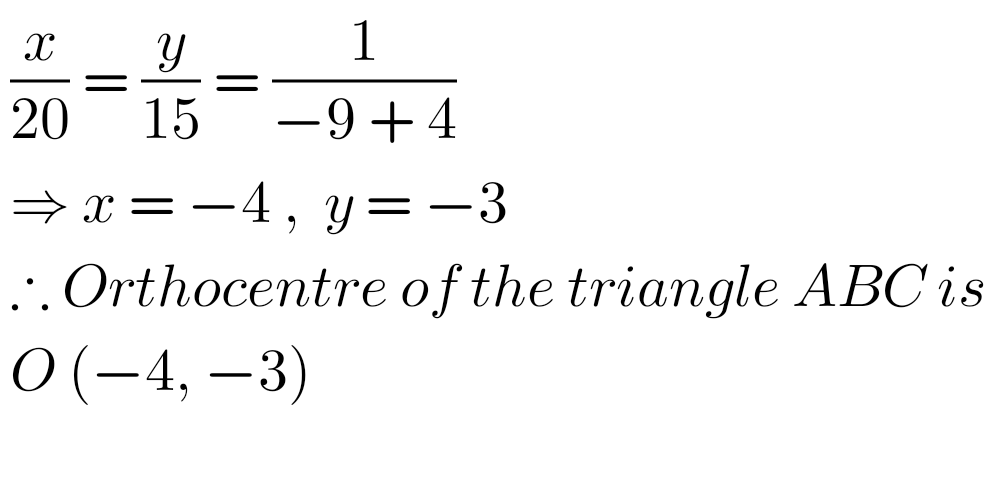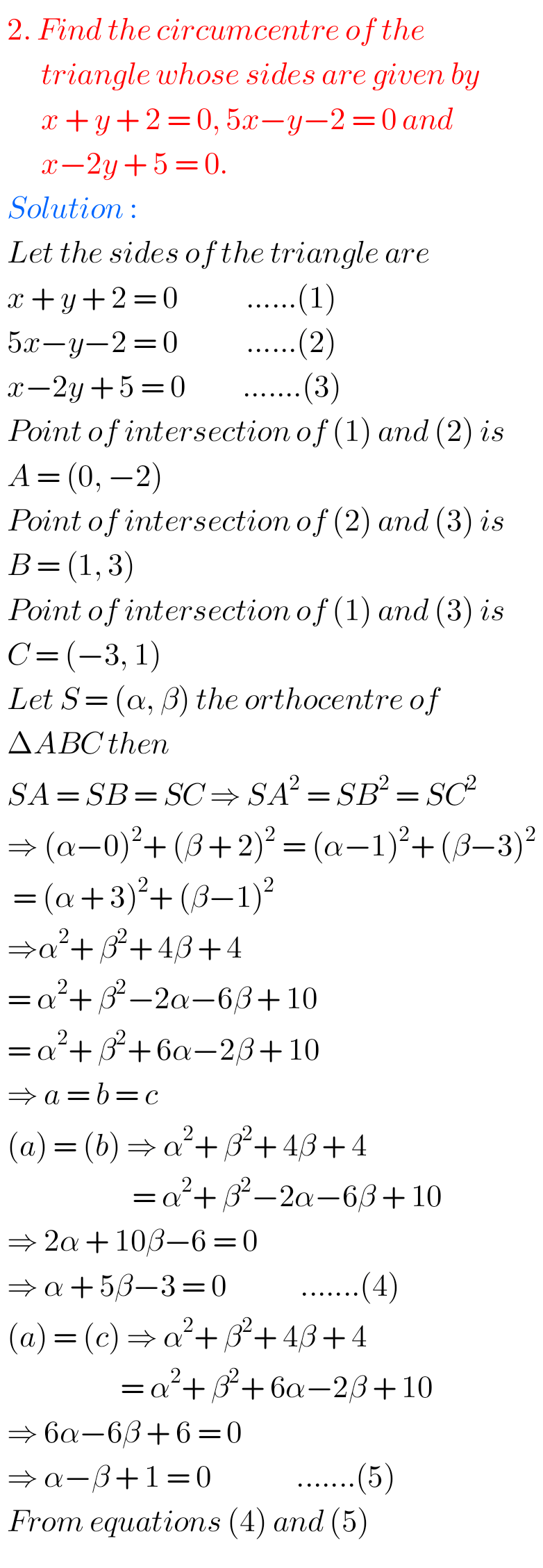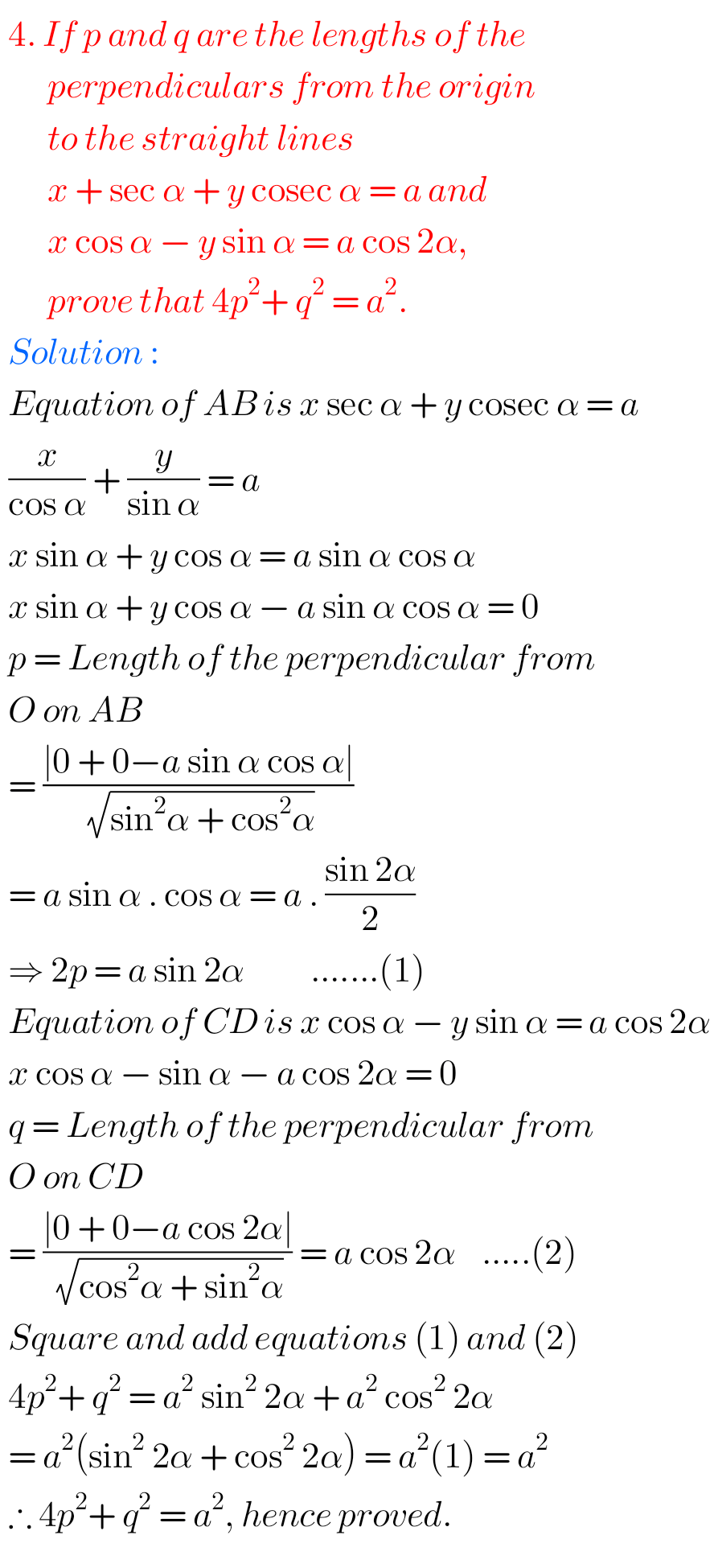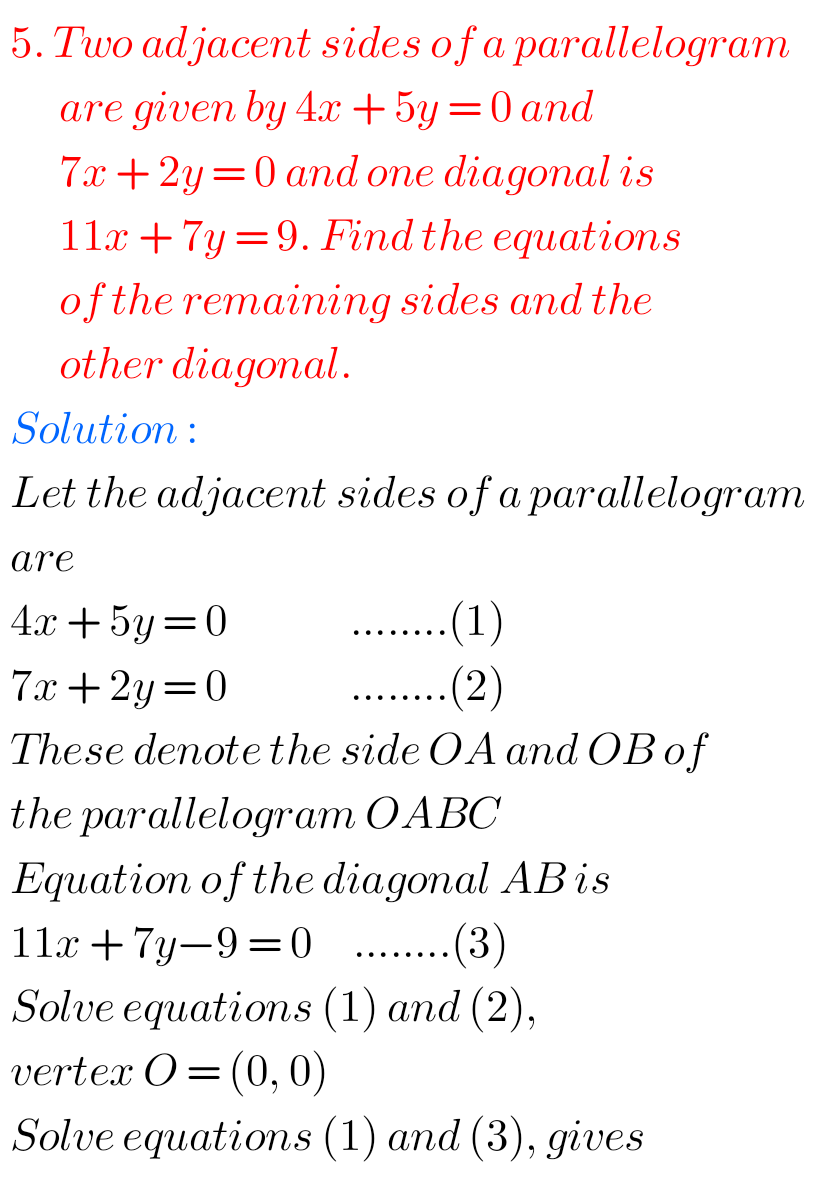F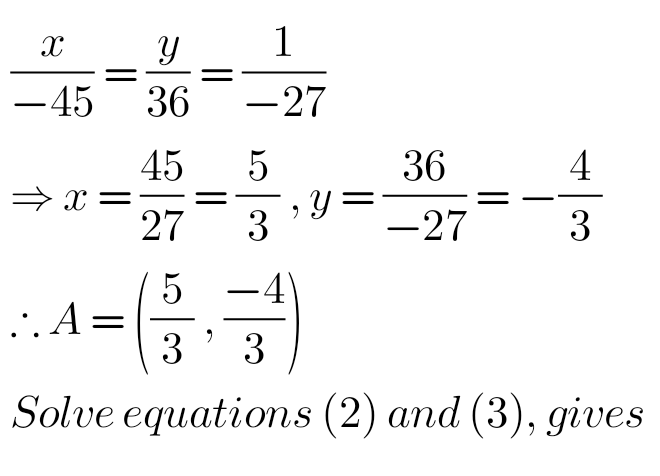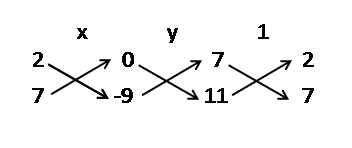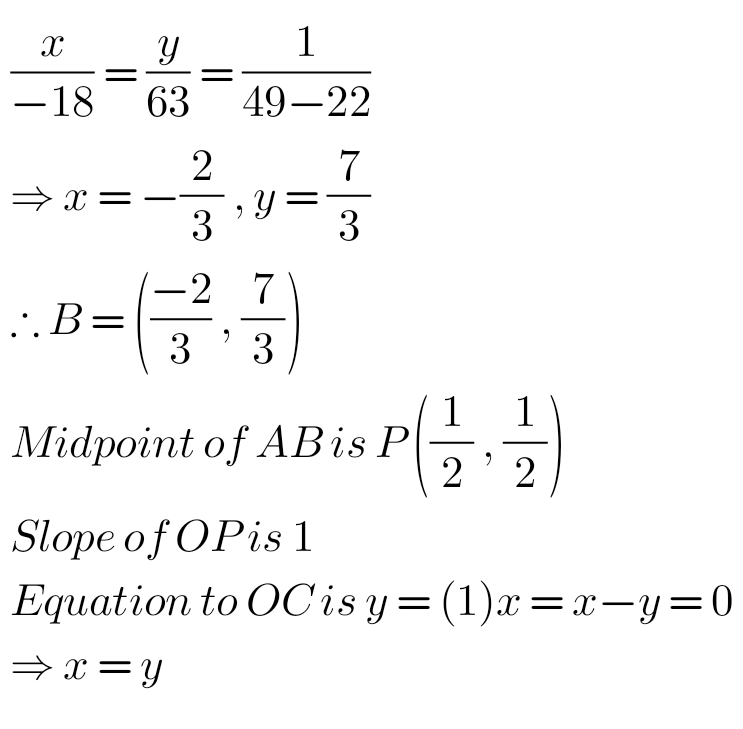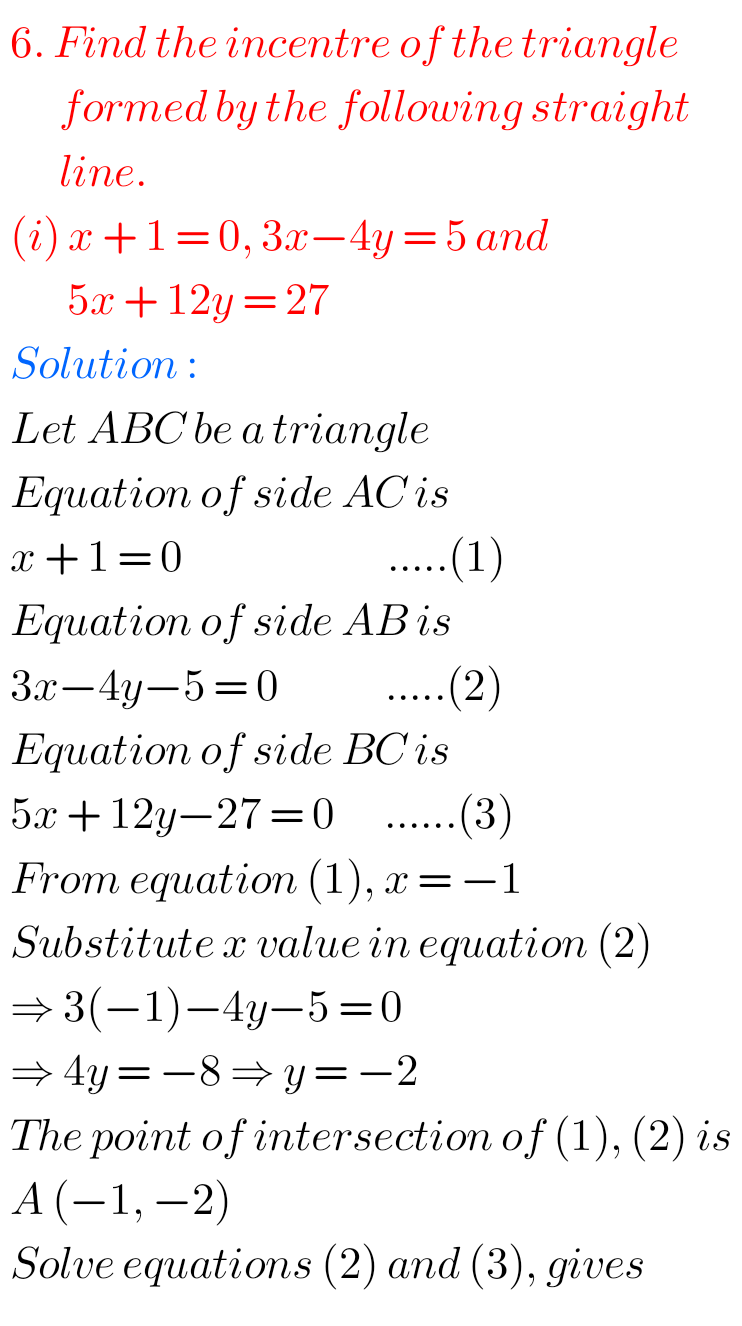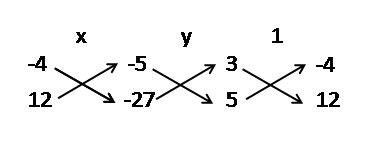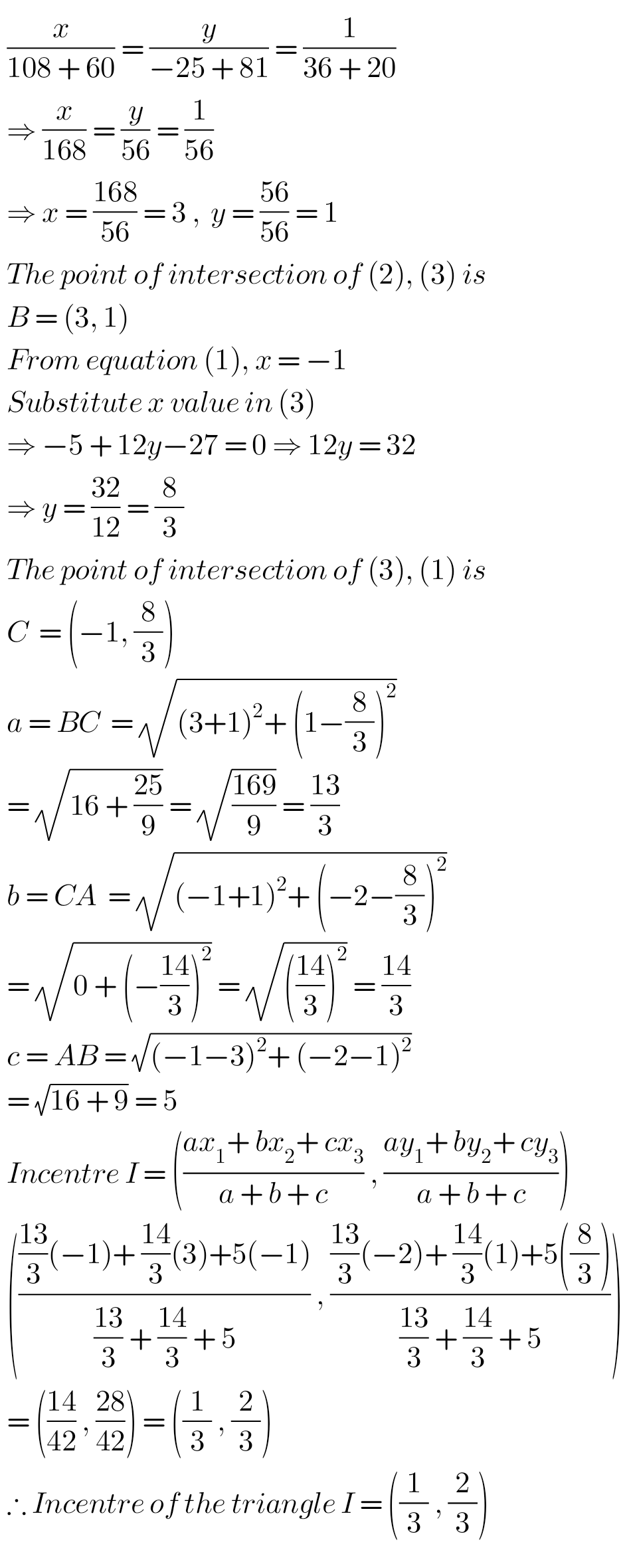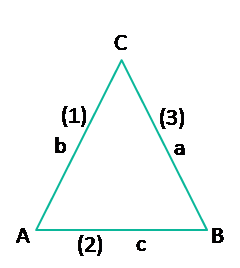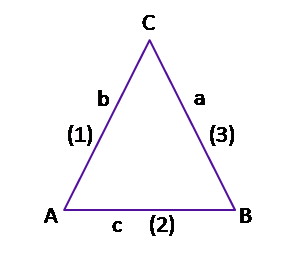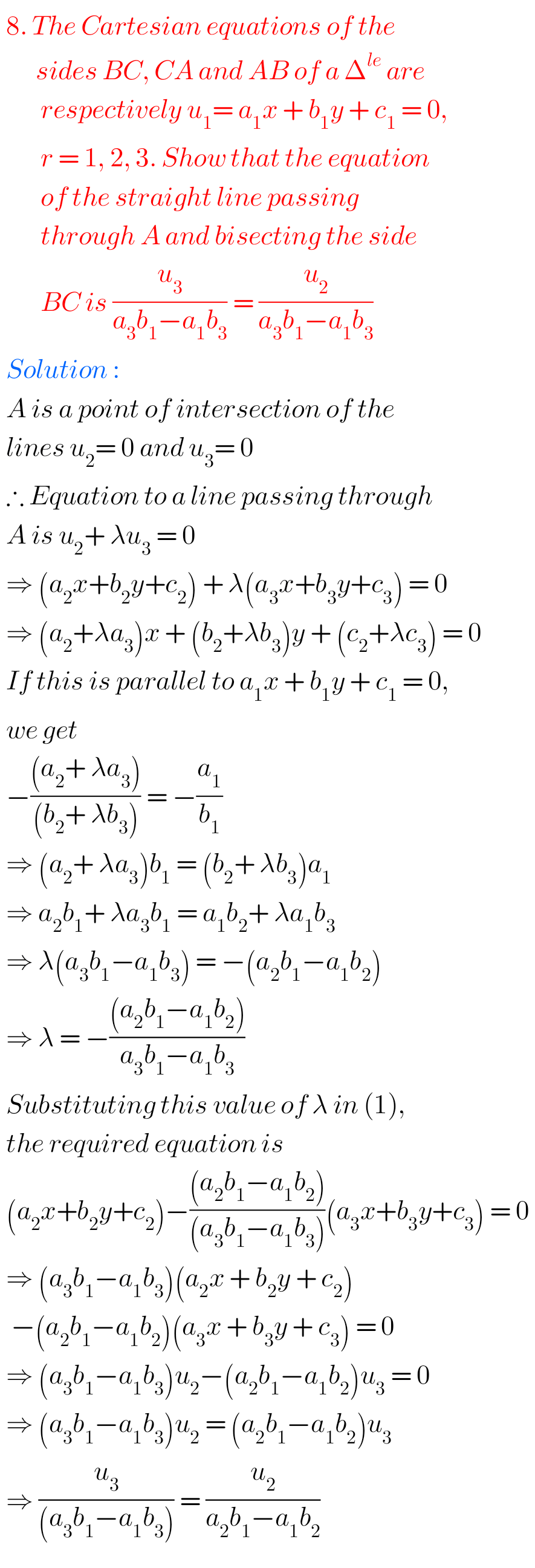M

Note : Observe the solutions and try them in your iwn method.